## Introduction

Gathering and storage of data of various kinds are activities that are of fundamental importance in all areas of science and engineering, social sciences and the commercial world. The amount of data being gathered and stored is growing at a phenomenal rate, because of the notion that the data can be used effectively to cure disease, recognize and mitigate social dysfunction and make businesses more efficient and profitable. In order to realize this promise, however, one must develop methods for understanding large and complex data sets in order to turn the data into useful knowledge. Many of the methods currently being used operate as mechanisms for verifying (or disproving) hypotheses generated by an investigator and therefore rely on that investigator to formulate good models or hypotheses. For many complex data sets, however, the number of possible hypotheses is very large and the task of generating useful ones becomes very difficult. In this paper, we will discuss a method that allows exploration of the data, without first having to formulate a query or hypothesis. While most approaches to mining big data focus on pairwise relationships as the fundamental building block1, here we demonstrate the importance of understanding the “shape” of data in order to extract meaningful insights. Seeking to understand the shape of data leads us to a branch of mathematics called topology. Topology is the field within mathematics that deals with the study of shapes. It has its origins in the 18th century, with the work of the Swiss mathematician Leonhard Euler2. Until recently, topology was only used to study abstractly defined shapes and surfaces. However, over the last 15 years, there has been a concerted effort to adapt topological methods to various applied problems, one of which is the study of large and high dimensional data sets3. We call this field of study Topological Data Analysis, or TDA. The fundamental idea is that topological methods act as a geometric approach to pattern or shape recognition within data. Recognizing shapes (patterns) in data is critical to discovering insights in the data and identifying meaningful sub-groups. Typical shapes which appear in these networks are “loops” (continuous circular segments) and “flares” (long linear segments). We typically use these template patterns in an informal way, then identify interesting groups using these shapes. For example, we might select groups to be the data points in the nodes concentrated at the end of a flare. These groups can then be studied with standard statistical techniques. Sometimes, it is useful to make a more formal definition of flares, when we would like to demonstrate by simulations that flares do not appear from random data. We give an example of this notion later in the paper. We find it useful to permit both the informal and formal approaches in our exploratory methodology.

There are three key ideas of topology that make extracting of patterns via shape possible. Topology takes as its starting point a metric space, by which we mean a set equipped with a numerical notion of distance between any pair of points. The first key idea is that topology studies shapes in a coordinate free way. This means that our topological constructions do not depend on the coordinate system chosen, but only on the distance function that specifies the shape. A coordinate free approach allows topology the ability to compare data derived from different platforms (different coordinate systems).

The second key idea is that topology studies the properties of shapes that are invariant under “small” deformations. To describe small deformations, imagine a printed letter “A” on a rubber sheet and imagine that the sheet is stretched in some directions. The letter will deform, but the key features, the two legs and the closed triangle remain. In a more mathematical setting, the invariance property means that topologically, a circle, an ellipse and the boundary of a hexagon are all identical, because by stretching and deforming one can obtain any of these three shapes from any other. The property that these figures share is the fact that they are all loops. This inherent property of topology is what allows it to be far less sensitive to noise and thus, possess the ability to pick out the shape of an object despite countless variations or deformations.

The third key idea within topology is that of compressed representations of shapes. Imagine the perimeter of the Great Salt Lake with all its detail. Often a coarser representation of the lake, such as a polygon, is preferable. Topology deals with finite representations of shapes called triangulations, which means identifying a shape using a finite combinatorial object called a simplicial complex or a network. A prototypical example for this kind of representation is the identification of a circle as having the same shape as a hexagon. The hexagon can be described using only a list of 6 nodes (without any placement in space) and 6 edges, together with data indicating which nodes belong to which edges. This can be regarded as a form of compression, where the number of points went from infinite to finite. Some information is lost in this compression (e.g. curvature), but the important feature, i.e. the presence of a loop, is retained.

Topological Data Analysis is sensitive to both large and small scale patterns that often fail to be detected by other analysis methods, such as principal component analysis, (PCA), multidimensional scaling, (MDS) and cluster analysis. PCA and MDS produce unstructured scatterplots and clustering methods produce distinct, unrelated groups. These methodologies sometimes obscure geometric features that topological methods capture. The purpose of this paper is to describe a topological method for analyzing data and to illustrate its utility in several real world examples. The first example is on two different gene expression profiling datasets on breast tumors. Here we show that the shapes of the breast cancer gene expression networks allow us to identify subtle but potentially biologically relevant subgroups. We have innovated further on the topological methods4,5 by implementing the idea of visually comparing shapes across multiple networks in the breast cancer case. The second example is based on 20 years of voting behavior of the members of the US House of Representatives. Here we show that the shapes of the networks formed across the years tell us how cohesive or fragmented the voting behavior is for the US House of Representatives. The third example is defining the characteristics of NBA basketball players via their performance statistics. Through these advanced implementations of topological methods, we have identified finer stratifications of breast cancer patients, voting patterns of the House of Representatives and the 13 playing styles of the NBA players.

## Results

### Mathematical underpinnings of topological data analysis (TDA)

TDA applies the three fundamental concepts in topology discussed in the introduction to study large sets of points obtained from real-world experiments or processes. The core problem addressed by TDA is how to use data sampled from an idealized space or shape to infer information about it. Figure 1 illustrates how our particular topological method based on a generalized Reeb graph6, operates on sampled points from a human hand. The method takes three inputs: a distance metric, one or more filter functions (real valued quantities associated to the data points) and two resolution parameters (“resolution” and “percent overlap”) and constructs a network of nodes with edges between them. The layouts of the networks are chosen using a force directed layout algorithm. As such, the coordinates of any individual node have no particular meaning. Only the connections between the nodes have meaning. Hence, a network can be freely rotated and placed in different positions with no impact on the interpretation of the results. The nodes represent sets of data points and two nodes are connected if and only if their corresponding collections of data points have a point in common (see the Methods section). The filter functions are not necessarily linear projections on a data matrix, although they may be. We often use functions that depend only on the distance function itself, such as the output of a density estimator or a measure of centrality. One measure of centrality we use later is L-infinity centrality, which assigns to each point the distance to the point most distant from it. When we do use linear projections such as PCA, we obtain a compressed and more refined version of the scatterplot produced by the PCA analysis. Note that in figure 1, we can represent a dataset with thousands of points (points in a mesh) in 2 dimensions by a network of 13 nodes and 12 edges. The compression will be even more pronounced in larger datasets.

The construction of the network involves a number of choices including the input variables. It is useful to think of it as a camera, with lens adjustments and other settings. A different filter function may generate a network with a different shape, thus allowing one to explore the data from a different mathematical perspective. Some filter functions may not produce any interesting shapes (such as a straight line). One works with a data set experimentally to find values for which the network structure permits the identification of subgroups (such as the tips of flares, or clusters) of interest.

### Applications of TDA in the real world

In order to show the implementation of TDA, we apply it to three very different data sets. We analyzed two disparate datasets of gene expression profiling data on breast tumors, 22 years of voting behavior of the members of the US House of Representatives and characteristics of NBA basketball players via their performance statistics. We show that the coordinate invariance and the related insensitivity to deformation are useful in reconciling the results from two distinct microarray studies. The innovation in this paper is to demonstrate that correspondences between multiple networks, whether it be over time, over disparate data sets or over changes of scale, are extremely important and can lead to novel insights. We discuss the parameters that go into the analyses, as well as the definition of filters related to the singular value decomposition, in the Methods section.

### Identifying patient subsets in breast cancer

The first application is the identification of patient sub-populations in breast cancer. We show here that topological maps can more finely stratify patients than standard clustering methods. We also identified interesting patient sub-groups that may be important for targeted therapy. Breast cancer continues to confound us, with multiple sub-types being identified to date. Identifying subtypes of cancer in a consistent manner is a challenge in the field since sub-populations can be small and their relationships complex. It is well understood that the expression level of the estrogen receptor gene (ESR1) is positively correlated with improved prognosis, given that this set of patients is likely to respond to standard therapies. However, among these high ESR1 patients, there are still sub-groups that do not respond well to therapy. It is also generally understood that low ESR1 levels are strongly correlated with poor prognosis although patients with low ESR1 levels but high survival have been identified over the years7. Many researchers have continued to find sub-groups that are enriched in different pathways8,9,10. Although many molecular sub-groups have been identified, it is often difficult to identify the same sub-group in a broader setting, where data sets are generated on different platforms, on different sets of patients and at a different times, because of the noise and complexity in the data11,12.

We use two relatively older breast cancer data sets, NKI13 and GSE203414, to demonstrate that even with older data sets, there is much to be gained by using this approach. The first data set, NKI, consists of gene expression levels extracted from 272 tumors and is analyzed using about 1500 most varying genes13,15,16. Although we are able to compute with any variance threshold, we show in Figure S1 that the shape in the network becomes less distinct as the threshold is relaxed. We therefore used the top most varying genes for this example. In addition to gene expression data, the NKI data set includes survival information. Figure 2 (panel A) shows the network constructed using both gene expression columns and survival information. We use correlation distance between gene expression vectors together with two filter functions, L-infinity centrality and survival variable (event death). The L-infinity centrality function captures the structure of the points far removed from the center or norm. The survival filter is used as a supervised step to study the behavior of the survivors separately from the non-survivors (filter functions described in Materials and Methods).

Note that the resulting network has a structure shaped like a horizontal letter Y along with several disconnected components. The patients that survived form the Y and the patients that did not survive form the smaller networks. The nodes of the network represent sets of tumors and are colored according to the average expression value of the ESR1 expression levels of those tumors. As mentioned earlier, low ESR1 levels often correspond to poor prognoses. It is interesting then to discover that the lower arm of the Y network of survivors is comprised entirely of tumors with low ESR1 expression levels (called lowERHS hereafter). In contrast, the low ESR1 non-survivors (lowERNS hereafter) are comprised of 3 smaller disconnected groups. Note that there is no need to determine a priori the threshold of ESR1 gene expression levels, which is often required by other methods when determining “ESR1 positive” or “ESR1 negative” status. The network was generated in an assumption-free manner. Mathematical validation of the data structures uncovered by TDA is described in Materials and Methods.

However, in order to corroborate that the lowERHS group is indicative of underlying biology, we performed the same analysis on the second breast cancer dataset, GSE203414. This dataset records the time to relapse instead of survival data. By obviating the need to transform the coordinates in the data matrices, we were able to visually compare topological maps constructed from the two data sets generated from entirely different experimental platforms. Once again, a very similar network was found (Figure 2, panel C). The large survivor/non-relapse structure comprised a horizontal letter Y with its lower arm defining a lowERHS group.

In order to test if these two lowERHS populations are enriched in some particular pathways, we performed a Kolmogorov-Smirnov test and identified a list of genes that best differentiated this sub-group from the rest of the structure17. These genes, including CCL13, CCL3, CXCL13 and PF4V1, are significantly enriched in the chemokine KEGG pathway (enrichment p-value 1.49E-4). When the nodes of these networks are colored by the average levels of these genes in the KEGG chemokine pathway, it was clear that both lowERHS sub-populations had higher than average values of the levels of these genes than the lowERNS (non survivor/relapse) group (see Table S1 for quantitative differences). In contrast, the excellent survival high ESR1 groups exhibit low chemokine levels. However, even though the chemokine pathway is on average higher in expression in lowERHS than in lowERNS, it is likely not the only determining factor. The low ERNS group is comprised of 3 smaller sub-networks and the chemokine activity varies between them, indicating that there are more sub-groups within the lowERNS.

In summary, the topological maps identified various sub-groups of breast cancer patients that are consistent between two different data sets. In particular, we have identified a group of surviving patients with low ESR1 expression occurring consistently across the two independent studies and for which the expression levels of genes in the immune pathways are elevated. We note that these subgroups are easily detected by our methods even across two disparate platforms because of the coordinate free property enjoyed by our approach. We show that classical single linkage hierarchical clustering approaches cannot easily detect these biologically relevant sub-groups (Figure 3) because by their nature they end up separating points in the data set that are in fact close.

### Implicit networks of the US House of Representatives based on voting behavior

The next data set to which we applied TDA is comprised of 22 years of voting records from the members of the US House of Representatives. The networks derived from the voting behavior of the members of the House differ from year to year, with some years having more sub-groups than others. We show that these sub-groups cannot be easily identified with methods such as PCA. We took the ‘aye’, ‘nay’ and ‘present but not voting’ votes of every member of the house for every year between 1990 to 2011 and built networks of the representatives based on their voting behavior for each year (figure S2). Details of the construction are provided in the Supplementary materials. The majority of the members either fall into the Republican group or the Democratic group with a small percentage of the members being independent or affiliated with either of the major party. Generally, every year the relationships between the members of the House based on voting patterns show that the Respublicans and the Democrats vote along party lines. However, upon closer inspection, there are many sub-groups within each political group. For some years, the relationships are more cohesive where there are large connected maps, but in other years, the networks show a very high degree of fragmentation. Figure 4 shows a plot of the fragmentation index that we have derived from the networks. The fragmentation index is computed by counting the number of connected components in the network (singletons excluded) for each year. This very high degree of fragmentation is evident in 2008 and 2010. There were many political issues that were voted on for these two years that could explain such fragmentation. In 2008, the US experienced a meltdown in the economy and in 2010, the healthcare bill was one of the most fiercely debated issue among many. We show that a PCA analysis of the same data was not able to show the fragmentation of voting behavior. As expected, the signal that was detected by PCA was the more obvious Republican and Democratic divide (figure S3). We also determined what issues are dividing the Republicans into the two main sub-groups (G1 and G2) in 2009. Among the top issues that most effectively divided these two Republican groups were The Credit Cardholders' Bill of Rights, To reauthorize the Marine Turtle Conservation Act of 2004, Generations Invigorating Volunteerism and Education (GIVE) Act, To restore sums to the Highway Trust Fund and for other purposes, Captive Primate Safety Act, Solar Technology Roadmap Act and Southern Sea Otter Recovery and Research Act. On these issues, the Republican subgroup (G2) was voting very similarly to the Democrats. Interestingly, this subgroup consisted of members that voted like another subgroup of Democrats on certain issues and persisted through the years (figure S4). We have termed these members the “Central Group”. This “Central Group” was coherent across many years with some core members like Sherwood Boehlert (R-NY) and Ike Skelton (D-MO) persisting over 10 years in such networks, while other members joined or dropped out across the years. Additional members of this group include Billy Tauzin (D/R-LA) and John McHugh (R-NY). These members of the House are often flagged as conservative Democrats or Liberal Republicans. This Central Group, even during the years when it broke away from each other along party lines, had weaker connections to the other networks of its own party. Again, this stratification is very difficult to locate using PCA analysis (figure S3).

The final dataset we studied is a data set that encodes various aspects of performance among basketball players in the National Basketball Association (NBA). Using rates (per minute played) of rebounds, assists, turnovers, steals, blocked shots, personal fouls and points scored, we identified more playing styles than the traditional five. The distance metric and filters used in the analysis were variance normalized Euclidean and principal and secondary SVD values, respectively. The positions in basketball are traditionally classified as guards, forwards and centers. Over time, this classification has been refined further into five distinct positions, namely point guard, shooting guard, small forward, power forward and center. These positions represent a spectrum of players from short, fast and playing outside the key to tall, slow and playing inside the key. However, distinguishing players based only on physical characteristics such as height or speed is perhaps arbitrary and outdated. One can then ask the question if there is instead a more informative stratification of player types based on their in-game performance. To answer this question, we constructed performance profiles for each of the 452 players in the NBA by using data from the 2010–2011 NBA season (Figure 5).

From the networks, we see a much finer structure than five distinct categories. These structures represent groups of players based on their in-game performance statistics. For example, the left side of the main network reveals a finer stratification of guards into three groups, namely offensive point guards, defensive point guards and ball handling shooting guards. We also see three smaller structures in the lower central part of the map that we labeled “All NBA” and “All NBA 2nd team”. The “All NBA” network consists of the NBA's most exceptional players and the second team consists of players who are also all-around excellent players but perhaps not as top-performing as the “All NBA” players. Within “All NBA” group are all-star players like LeBron James and Kobe Bryant. Interestingly, there are some less well-known players in the “All NBA” network such as Brook Lopez, suggesting that they are potential up and coming stars. It is of note that the “All NBA” and “All NBA 2nd team” networks are well separated from the large network, indicating that their in-game statistics are very different. To also illustrate the capability to perform multi-resolution analyses simultaneously on the same dataset and how that kind of analysis is important, we compared the high resolution network (Figure 5, right panel) to the lower resolution network (Figure 5, left panel). The right panel shows that at a lower resolution, these players form 4 categories, which are scoring big men, paint protectors, scoring guards and ball handling guards. In summary, this topological network suggests a much finer stratification of players into thirteen positions rather than the traditional division into five positions.

## Discussion

We have shown that TDA can handle a variety of data types using three real world examples. The three key concepts of topological methods, coordinate freeness, invariance to deformation and compressed representations of shapes are of particular value for applications to data analysis. Coordinate free analysis means that the representation is independent of the particular way in which the data set is given coordinates, but rather depends only on the similarity of the points as reflected in the distance function. Coordinate free representations are vital when one is studying data collected with different technologies, or from different labs when the methodologies cannot be standardized. The invariance to deformation provides some robustness to noise. Compressed representations are obviously important when one is dealing with very large data sets, but even for moderate size data sets they provide more succinct and understandable representations than most standard methods. Finally, we have shown that our novel implementation of the idea of correspondence between multiple networks, whether it be over time or disparate data sets, or over changes of scale is extremely important and can lead to novel insights. The usefulness of TDA is not restricted to these three types of applications but can generally be applied to many different data types, including nucleic acid sequencing reads for de novo assembly, structure of interactions among people, unstructured text, time series data, reconstruction of metagenomes represented in complex microbiome communities and others.

## Methods

### TDA Pipeline

The two resolution parameters (a number N of intervals and p, a percent overlap), determine a collection of N intervals of equal length with a uniform overlap of p percent of the length of the intervals. The real world data set here is a sampling of points from a 3D mesh that represents the shape of hand (Figure 1A). The metric is three-dimensional Euclidean distance and the filter function is the x-coordinate (the set is colored by the filter function values). By using the collection of intervals specified above, the data set is binned into groups, whose filter values lie within a single interval, giving rise to a collection of overlapping bins (figure 1C). Note that since we chose the intervals to be overlapping, the binned data represents a systematic oversampling of the original data. We frequently use filter functions which depend explicitly only on the distance function used and not on the representation of the data as a data matrix. Examples of such functions would be various proxies for distance-based density, measures of centrality and various coordinates of a multidimensional scaling analysis. Such filters are called geometric filters and are quantities that are important in any statistical analysis. One particular such filter, L-infinity centrality, is defined for a data point x to be the maximum distance from x to any other data point in the set. Large values of this function correspond to points that are far from the center of the data set. It is also useful to use some filters that are not geometric, in that they do depend on a coordinate representation, such as coordinates in principal component or projection pursuit analysis. As we will demonstrate, when our construction is performed using such filters, it will produce a more detailed and also more succinct description of the data set than the scatter plots that are typically displayed.

The final step in our pipeline is to apply a clustering scheme to each bin. We apply single linkage clustering, but other clustering schemes would also work. Let N be the number of points in a bin. We first construct the single linkage dendrogram for the data in the bin and record the threshold values for each transition in the clustering. We select an integer k and build a k-interval histogram of these transition values. The clustering is performed using the last threshold before the first gap in this histogram. The reason for this choice is that one frequently observes experimentally that the shorter edges which connect points within each cluster have a relatively smooth distribution and the edges which are required to merge the clusters are disjoint from this in the histogram. Note that larger values of k produce more clusters, smaller values fewer clusters. Occasionally the distribution of a variable is such that the binning process produces an excessively skewed histogram. In this case, the values are renormalized so as to make the distribution even and we call this process “equalized”. The clustering scheme thus partitions each bin into a list of partial clusters. Finally, we build a network whose nodes are the partial clusters. We connect two partial clusters with an edge if they have one or more data points in common (figure 1D). If we use two filter functions, we will need to choose families of intervals for each, to obtain a two dimensional array of rectangles instead of intervals. In this case, we might obtain three or four fold intersections that will give rise to triangles and tetrahedra. The choice of families of intervals corresponds to a level of resolution of the construction. A larger number of smaller intervals yields a network with more nodes and more edges and can be viewed as a higher resolution version of the construction. We note that our method provides a way to take advantage of the best properties of two different strands in data analysis, namely clustering and scatterplot methods such as principal component analysis, projection pursuit and multidimensional scaling18,19,20.

Our TDA pipeline is also highly parallelizable, which permits the interactive analysis of large data sets. As an example, the network construction for a synthetic dataset (will be downloadable as a part of supplementary materials) represented by a point cloud of 1 million rows and 50 columns takes 87 seconds to compute. It also permits us to study data sets without computing all possible pairwise distances. Although the role compression plays in pure topology is already mirrored in existing non-topological methods of data analysis, these methods such as a scatterplot, do so by simply creating a list of points of the same length as the original set. In this case, a scatter plot will still result in 1 million rows but with 2 columns. In contrast, our approach produces a network of 896 nodes and 897 edges.

### Mathematical validation of data structures uncovered by TDA

We describe a method for “validating” the presence of a flare in a data set, i.e. for determining that no flare could be obtained if the data is selected from a multivariate Gaussian distribution. To test the significance of flares we generated 1000 datasets of the same dimensionality as original data. The entries in each column of newly created datasets were drawn from Gaussian distribution with zero mean and constant variance across all columns. For each dataset we produce the associated graph using our methodology and apply to it a flare detection algorithm described below. Using this algorithm we count the number of flares found and compare it to the number of flares in the graph of the original data.

Let us now describe flare detection algorithm in detail. The first step is to compute an eccentricity function e(n) for each node of the graph: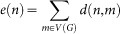where V(G) is the vertex set of the graph and d is the graph distance on the unweighted graph G. The intuition behind this choice is that such function should differentiate between nodes in central denser regions and nodes at the ends of flares. We order nodes in each connected component by their eccentricity value in decreasing order, let us denote this ordered list by L. Next, we set up a zero-dimensional persistence mechanism for each connected component of the graph using eccentricity as a persistence parameter. The persistence algorithm proceeds as follows. We process each node in L and at each moment we keep the following data: the set of connected components formed by nodes processed so far, the birth and death times for each component. The birth time is defined as the eccentricity value of the node which started the component (this is always the node with largest eccentricity value among all nodes in the component). The death time is defined as eccentricity value of the node which merges this component with another component with a higher birth value (until such merge happens the death time is set to 0). With addition of a node three things can happen. The node can (1) start a new component that will be created in case when none of its graph neighbors are processed yet, (2) can be added to an already existing component that will be created if precisely one of its graph neighbors was already processed, or (3) merge two or more components that will be created if two or more of node's graph neighbors were already processed and they belong to two or different components. After we traverse all the nodes in L, we have a set of connected components together with a pair of birth and death times for each. For each component we compute the absolute value of the difference between the death and birth values divided by the range of eccentricity values. The resulting value, which we will denote by V is in the interval [0,1]; in general, higher values of V correspond to those components which were started early, i.e. by higher eccentricity nodes and hence should correspond to flares.

Finally, for each x in [0,1] we count the number of components C such that V(C) < x. This defines a non-increasing integer-valued function. We identify the longest interval over which this function stays constant and greater than 1. We declare the corresponding components as flares. The output of the algorithm is the number of flares found by this procedure. This algorithm is applied to every connected component of a graph provided that this component is large enough in the number of nodes relative to the total number of nodes in the graph (the size threshold is set to 10% of graph size). This method, applied to randomly generated data as described above, did not produce more than one flare except once in 1000 Monte Carlo simulations.

### Mathematical validation of a patient group in the Y structure

In using the TDA methodology, one often colors the output network by a quantity of interest and this is useful in deriving actionable consequences of the analysis. For example, in a microarray study of cancer patients, one might color each node by the proportion of patients within that node who died within the period of the study. In earlier analyses, we have found connected families of adjacent nodes in which the survival is perfect, i.e. all patients survived the length of the study. The question arises, though, if this observation is an artifact of the TDA network construction. One can address this question as follows. Every microarray data set gives rise to a finite metric space, since one can assign a number of choices of distances to the rows of the data matrix. For any finite metric space X, one can associate a family of proxies for density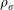on X parameterized by a bandwidth parameter, by the formula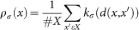where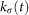is a probability density function such as the Gaussian distribution centered at the origin and with variance equal to. The quantity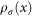is a proxy for the density within the metric space at the point x. We can consider an analogous distribution based only on the set L of patients who survive the study, to construct a density function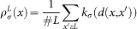which is a proxy for density of live patients close to a point x. The quotient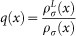now represents the relative density of live patients compared to the density of all patients in the metric space. A high value for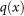indicates increased percentage of survival in this region of X. One will then want to find methods for assessing how high a value of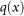is statistically significant. To perform such an analysis, we will assume as a null hypothesis that the set L is obtained by random selection from X. One can then select sets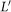uniformly at random, of the same cardinality as L and construct the corresponding quotients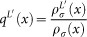We then perform a Monte Carlo simulation by repeated selection of sets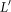and record the values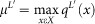. The distribution of these values gives us a criterion for determining how exceptional a given value of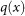is. Applied to a high survival flare5S, this value was found to occur with likelihood less than 10−4.

### Principal metric SVD filters

When data points are given in a matrix, one can apply the standard singular value decomposition to the data matrix to obtain subspaces within the column space and dimensionality reduction is accomplished by projection on these subspaces. The values of each of these coordinates can then be used as a filter for an analysis. When the data is simply given as a distance matrix, we produce a data matrix by assigning to each data point its column vector of distances to all the other data points. We then apply the standard singular value decomposition to this data matrix. This is done with standard linear algebraic techniques when possible and when the number of points is too large, numerical optimization techniques are used. Typically only the first and second singular vectors are used.

### Filter functions

Wherever required, we used the Pearson correlation between two tumors across all the chosen genes as the distance metric. Two filter functions were used for the breast cancer network construction. The first filter function is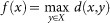This computes for every data point the maximal distance to any other data point in the set (L-infinity centrality). The second filter function was simply the binary variable representing survival.

### Datasets and software

Access to any of the three datasets via a trial license of the software can be requested by writing to the corresponding authors.